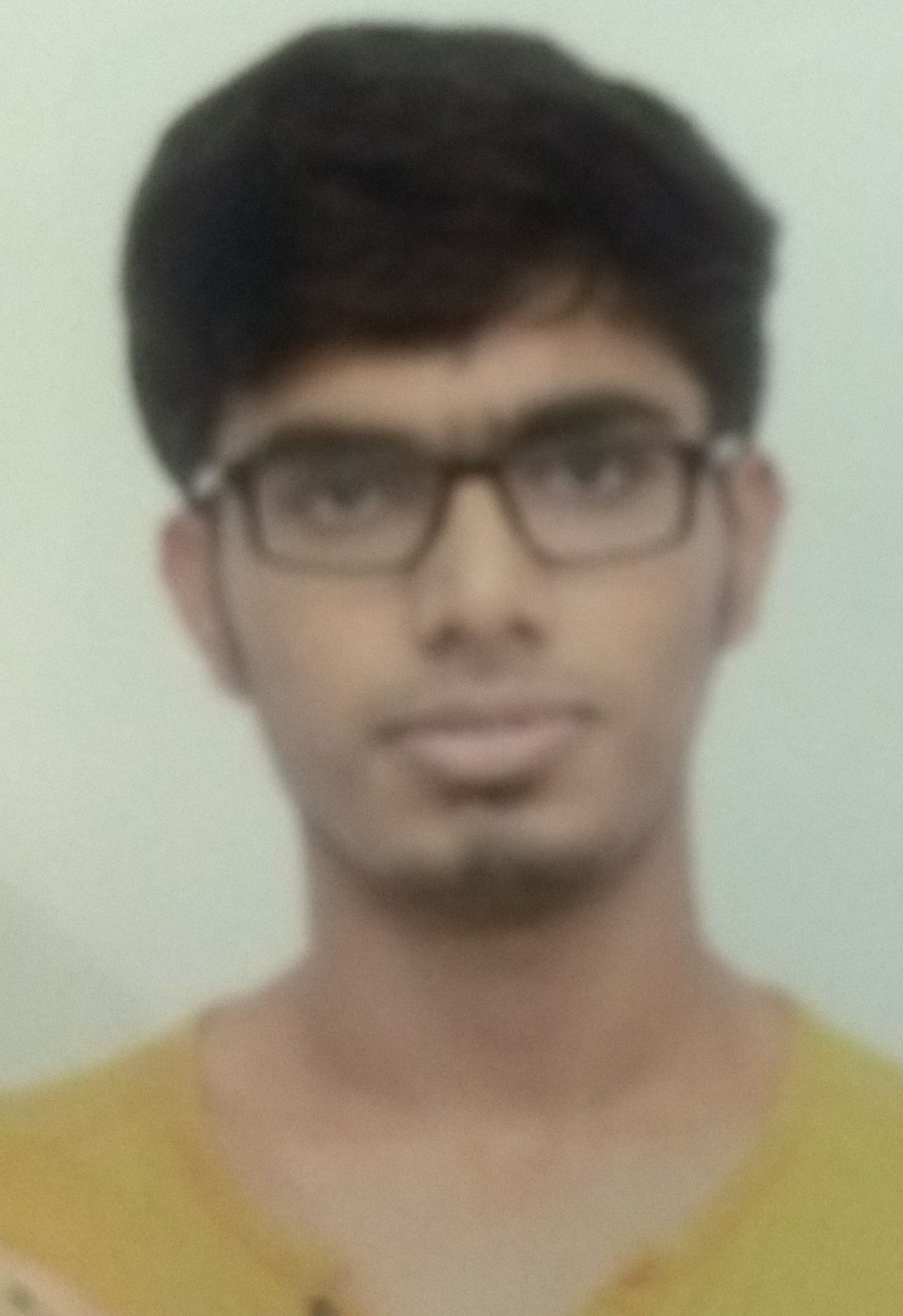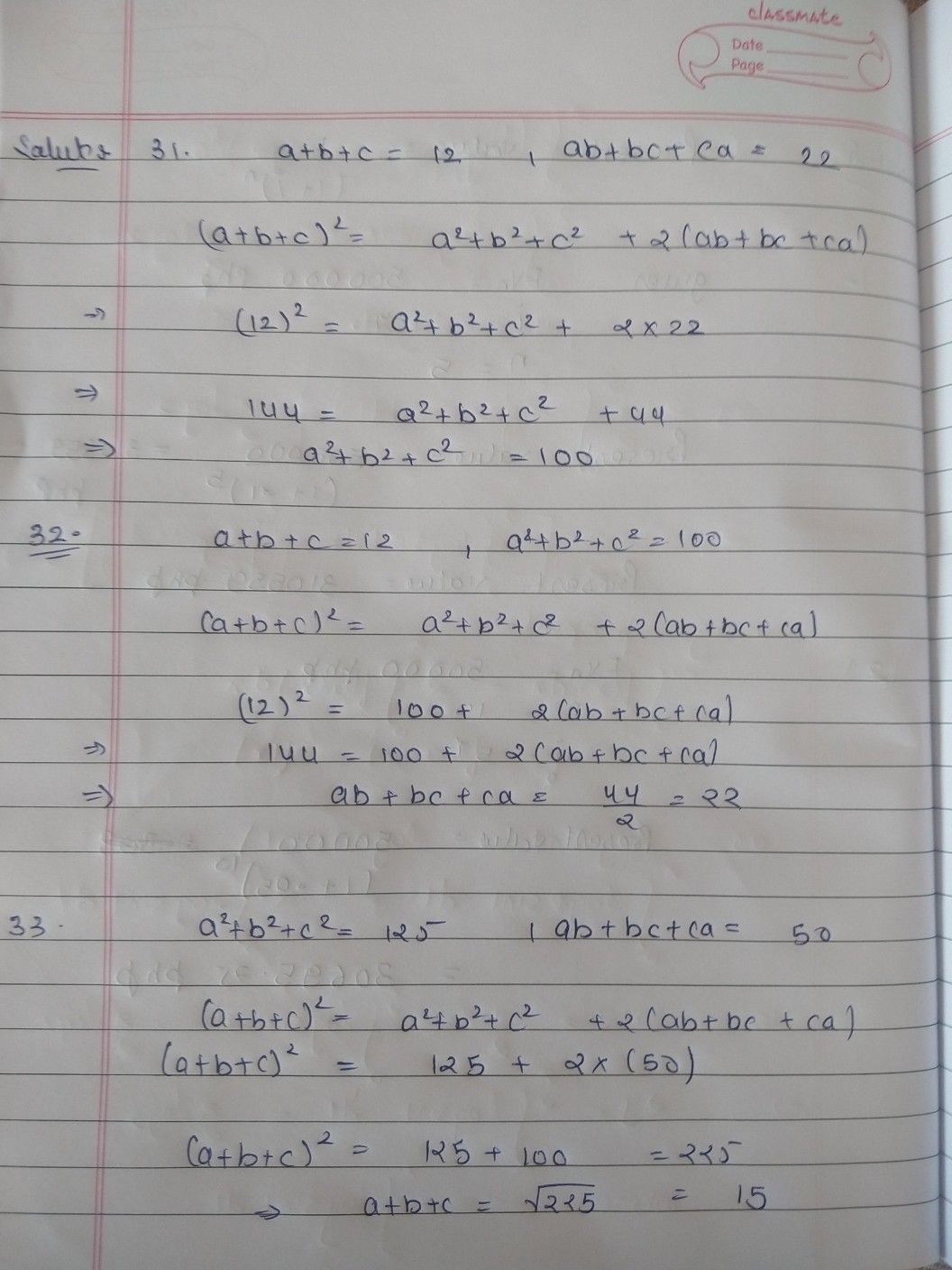Symbol
Problem$31$ If $a+b+c=12$ and $ab+bc+ca=22$ find $a^{2}+b^{2}+c^{2}$ $32$ If $a+b+c=12$ and $a^{2}+b^{2}+c^{2}=100$ find ab $+bc+ca$ $33$ If $a^{2}+b^{2}+c^{2}=125$ and ab $+bc+ca=50$ find a $4b⊥$
7th-9th grade
Algebra
Search count: 107
Question content
sum number 32
SolutionQanda teacher - nrohitTell me if you find any problem.Student
sir why you have done all the sumQanda teacher - nrohit
sorry I didn't notice that you only asked 32